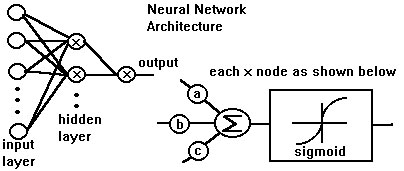## What are Neural Networks?Neural networks are a mathematical development which, in effect, allows a computer to perform learning with relatively minor human guidance and skill. The basic idea is to learn by example. Historical examples of market behavior and indicators are simply presented to the neural netwok and are used by the network to learn the relationship of indicators to future market behavior. The result of this learning process is a mathematical algorithm providing an estimate of future market behavior based on past values of indicators. Once the algorithm has been constructed, current values of the indicators are input and an estimate of market behavior tomorrow or next week are predicted. The basic architecture of a neural network algorithm is shown in the figure below. It consists of nodes, referred to as neurons in network parlance. It has an input neuron layer, output layer and one or more hidden layers. The input layer just represents the input indicators with each input being a current or a past value of a financial indicator. The output node represents the market prediction while the hidden layer provides the capability to transform the current indicator values into a prediction of future market behavior. Notice that nodes marked as "x" in the diagram consist of a summing junction and a sigmoid nonlinearity. The sigmoid non linearity is the key to the learning power of a neural network and allows it to learn complex relationships between indicators and market behavior. The learning process consists of determining the weighting constants a, b, and c. This is often done employing the back propagation algorithm which examines the historical examples and adjusts the constants. The potential of neural netwoks is startling as decribed in the famous Kolmogorov theorem. At a simplistic level, that theorem states that a neural net having a single hidden layer can represent ANY mathematical function. Even mathematicians are surprised by the power of this statement when first encountering it.